基于keil4和proteus实现的简易密码锁

1. 能设定一组4位的数字开启密码（设定密码功能）
2. 用LED小灯代替锁开启显示，输入密码正确，则小灯亮起（开锁功能）
3. 如果3次密码错误，则进行鸣叫报警，并在1分钟之内不能再次输入（报警功能）
4. 密码输入显示在数码管上，输入正确显示on，输入错误显示err（显示功能）
5. 可在以上功能上扩展。
二、原理说明
该程序用C51语言，Keil5软件及proteus进行仿真。
用到的硬件设备有AT89C52，蜂鸣器，LCD1602，上拉电阻，三极管，按钮
代码部分对不同的方法进行封装，主要是延时函数Delay(),蜂鸣器发声函数Beep(),矩阵键盘函数，LCD1602显示函数以及主要功能实现main函数。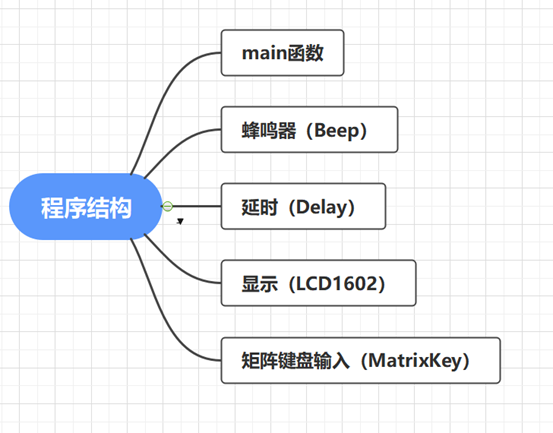1、 矩阵键盘输入

#include <REGX52.H>
#include "Delay.h"

unsigned char MatrixKey()
{
unsigned char KeyNumber = 0;
//按列扫描
P1 = 0xFF;
P1_3 = 0;  //判断第一列
if(P1_7 == 0){Delay(20);while(P1_7 == 0){Delay(20);KeyNumber = 1;} } //delay消抖，一列一行
if(P1_6 == 0){Delay(20);while(P1_6 == 0){Delay(20);KeyNumber = 5;} } //1列2行
if(P1_5 == 0){Delay(20);while(P1_5 == 0){Delay(20);KeyNumber = 9;} } //1列3行
if(P1_4 == 0){Delay(20);while(P1_4 == 0){Delay(20);KeyNumber = 13;} } //1列4行

P1 = 0xFF;
P1_2 = 0;  //判断第2列
if(P1_7 == 0){Delay(20);while(P1_7 == 0){Delay(20);KeyNumber = 2;} } //delay消抖，2列一行
if(P1_6 == 0){Delay(20);while(P1_6 == 0){Delay(20);KeyNumber = 6;} } //2列2行
if(P1_5 == 0){Delay(20);while(P1_5 == 0){Delay(20);KeyNumber = 10;} } //2列3行
if(P1_4 == 0){Delay(20);while(P1_4 == 0){Delay(20);KeyNumber = 14;} } //2列4行

P1 = 0xFF;
P1_1 = 0;  //判断第3列
if(P1_7 == 0){Delay(20);while(P1_7 == 0){Delay(20);KeyNumber = 3;} } //delay消抖，3列一行
if(P1_6 == 0){Delay(20);while(P1_6 == 0){Delay(20);KeyNumber = 7;} } //3列2行
if(P1_5 == 0){Delay(20);while(P1_5 == 0){Delay(20);KeyNumber = 11;} } //3列3行
if(P1_4 == 0){Delay(20);while(P1_4 == 0){Delay(20);KeyNumber = 15;} } //3列4行

P1 = 0xFF;
P1_0 = 0;  //判断第4列
if(P1_7 == 0){Delay(20);while(P1_7 == 0){Delay(20);KeyNumber = 4;} } //delay消抖，4列一行
if(P1_6 == 0){Delay(20);while(P1_6 == 0){Delay(20);KeyNumber = 8;} } //4列2行
if(P1_5 == 0){Delay(20);while(P1_5 == 0){Delay(20);KeyNumber = 12;} } //4列3行
if(P1_4 == 0){Delay(20);while(P1_4 == 0){Delay(20);KeyNumber = 16;} } //4列4行

return KeyNumber;
}

2、 LCD1602进行显示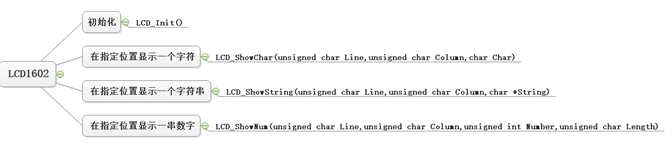3、 延时函数

void Delay(unsigned int xms)
{
unsigned char i, j;
while(xms--)
{
i = 2;
j = 239;
do
{
while (--j);
} while (--i);
}
}

4、 蜂鸣器

#include <REGX52.H>
#include "Delay.h"

sbit beep = P2^2;
void Beep()
{
beep = ~beep;
Delay(500);
}

5、 main函数
main函数是对整个电子密码锁主要逻辑的实现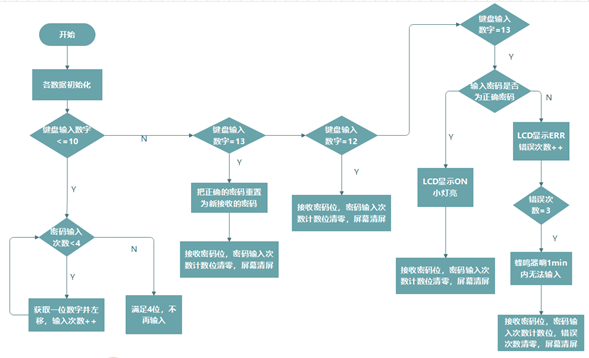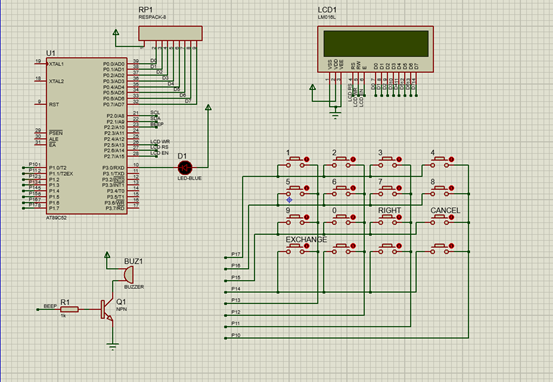AT89C52的P0口和P2口的P25,P26,P2^7控制LCD1602，P0口需接上拉电阻
AT89C52的P1口控制矩阵键盘。这里矩阵键盘按列扫描，首先对P1进行初始化P1 = 0xFF，然后循环依次从第一列到第四列的接口赋值0（P10,P11,P12,P13），然后对行的接口进行扫描检测。如果某一个按键按下，此时该按键列的接口为0，那么该按键行的接口也为0。根据行与列判断是哪一个按键进行相应赋值定义。
AT89C52的P3^0控制LED小灯。
AT89C52的P22控制蜂鸣器。蜂鸣器分为有源蜂鸣器和无源蜂鸣器（振荡源），我选择的为有源蜂鸣器，将蜂鸣器的电压调为2v。我用的NPN三极管，P22=1时三极管导通，蜂鸣器发声。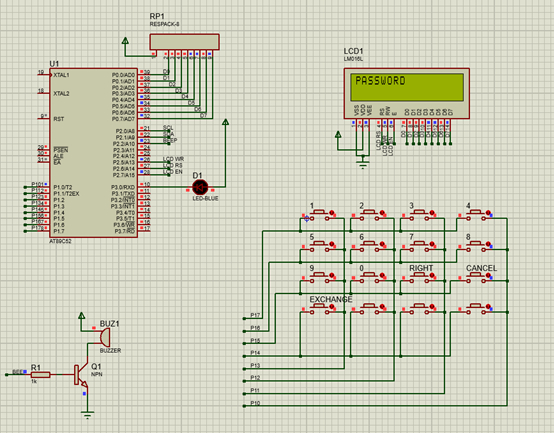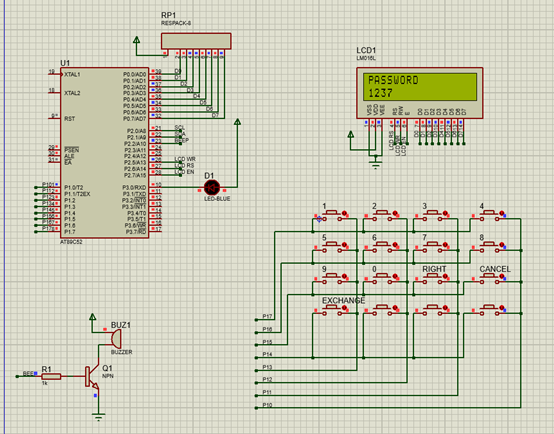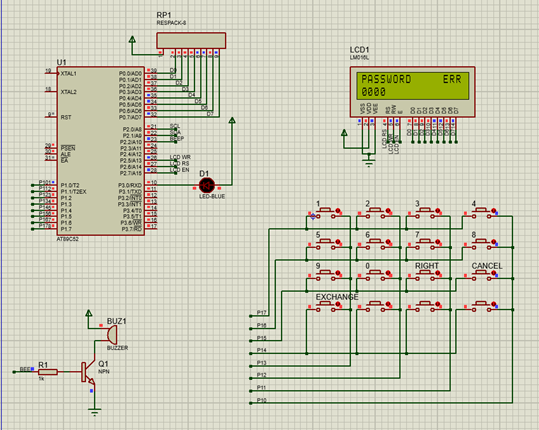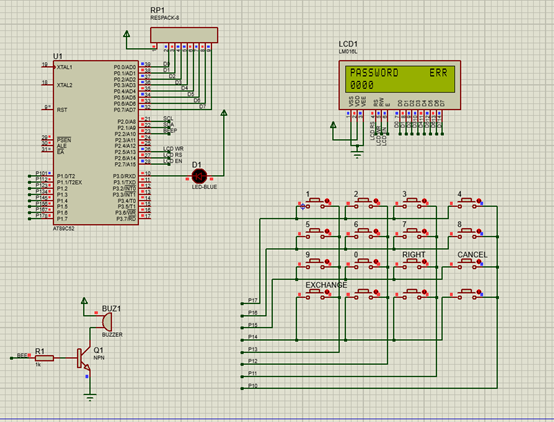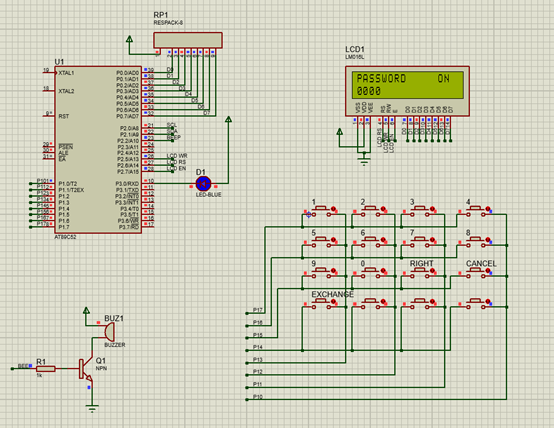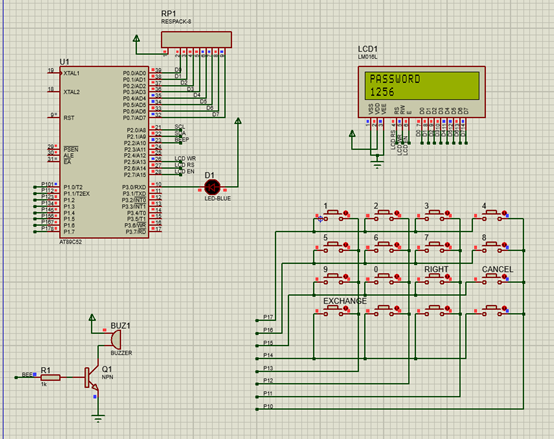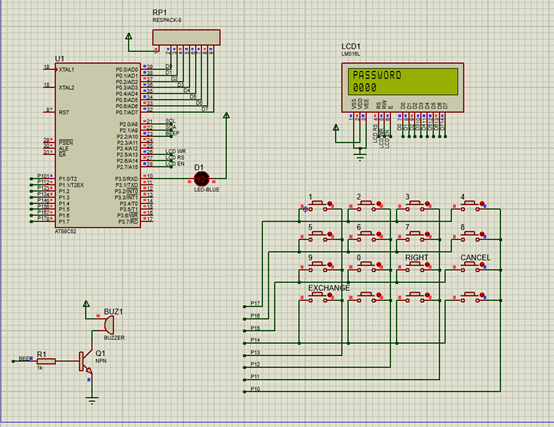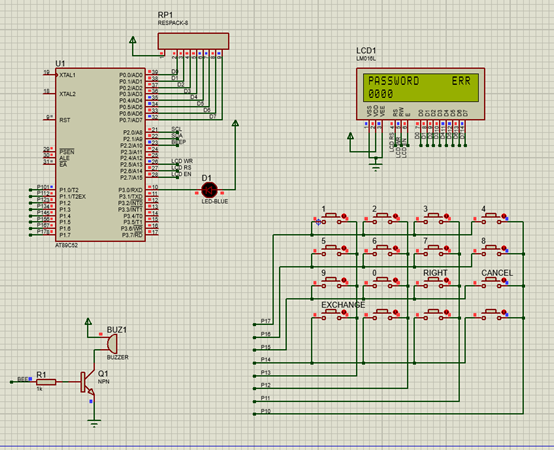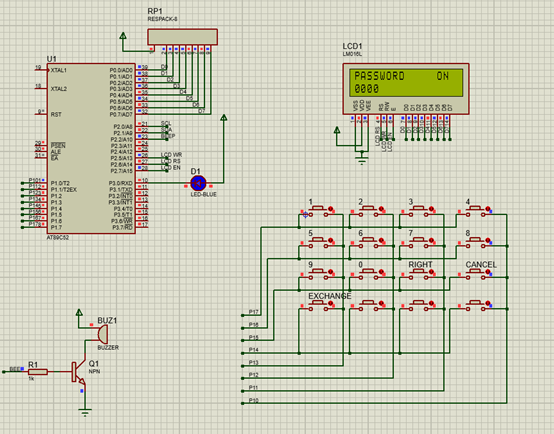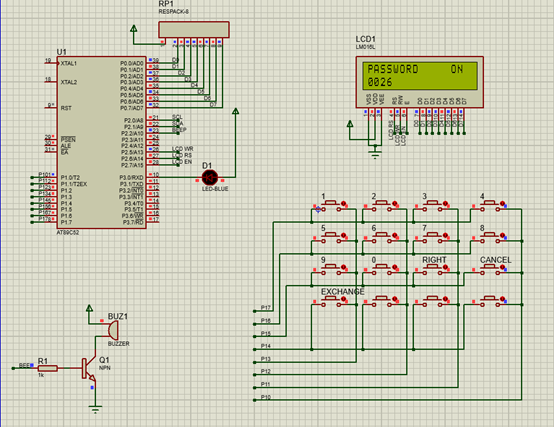04-1808-11
02-151万+
08-068163
03-05
03-173241
08-01
05-18765
11-242290
01-042739
06-137460
12-08134
05-25467¥2 ¥4 ¥6 ¥10 ¥20余额支付 (余额：-- )扫码支付获取中扫码支付点击重新获取扫码支付1.余额是钱包充值的虚拟货币，按照1:1的比例进行支付金额的抵扣。
2.余额无法直接购买下载，可以购买VIP、C币套餐、付费专栏及课程。余额充值